# Uniform honeycomb

In geometry, a uniform honeycomb or uniform tessellation or infinite uniform polytope, is a vertex-transitive honeycomb made from uniform polytope facets. All of its vertices are identical and there is the same combination and arrangement of faces at each vertex. Its dimension can be clarified as n-honeycomb for an n-dimensional honeycomb.

An n-dimensional uniform honeycomb can be constructed on the surface of n-spheres, in n-dimensional Euclidean space, and n-dimensional hyperbolic space. A 2-dimensional uniform honeycomb is more often called a uniform tiling or uniform tessellation.

Nearly all uniform tessellations can be generated by a Wythoff construction, and represented by a Coxeter–Dynkin diagram. The terminology for the convex uniform polytopes used in uniform polyhedron, uniform 4-polytope, uniform 5-polytope, uniform 6-polytope, uniform tiling, and convex uniform honeycomb articles were coined by Norman Johnson.

Wythoffian tessellations can be defined by a vertex figure. For 2-dimensional tilings, they can be given by a vertex configuration listing the sequence of faces around every vertex. For example 4.4.4.4 represents a regular tessellation, a square tiling, with 4 squares around each vertex. In general an n-dimensional uniform tessellation vertex figures are define by an (n-1)-polytope with edges labeled with integers, representing the number of sides of the polygonal face at each edge radiating from the vertex.

## Examples of uniform honeycombs

2-dimensional tessellations
Spherical Euclidean Hyperbolic

Main article: Uniform polyhedron
Coxeter diagramPicture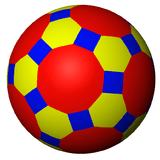Truncated icosidodecahedron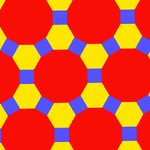Truncated trihexagonal tiling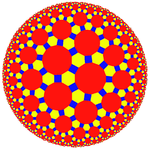Truncated triheptagonal tiling
(Poincaré disk model)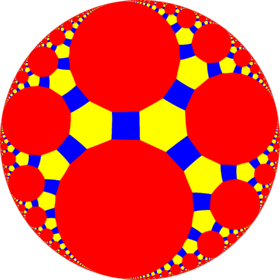Truncated triapeirogonal tiling
Vertex figure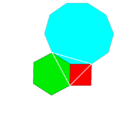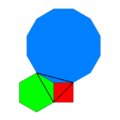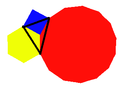3-dimensional honeycombs
3-spherical 3-Euclidean 3-hyperbolic

Main article: Uniform polychoron
and paracompact uniform honeycomb
Coxeter diagramPicture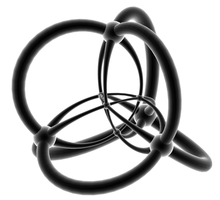(Stereographic projection)
16-cell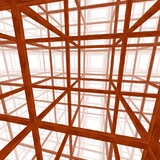cubic honeycomb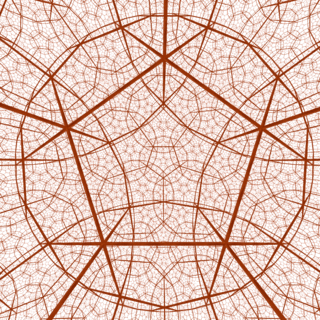order-4 dodecahedral honeycomb
(Beltrami–Klein model)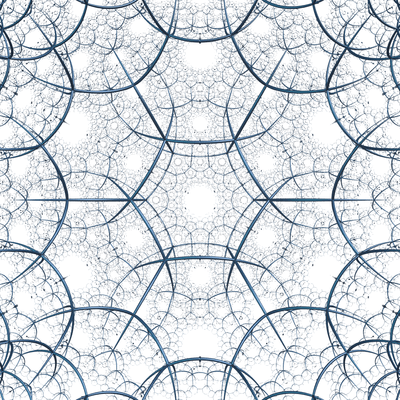order-4 hexagonal tiling honeycomb
(Poincaré disk model)
Vertex figure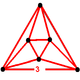(Octahedron)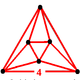(Octahedron)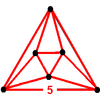(Octahedron)(Octahedron)

## References

• George Olshevsky, Uniform Panoploid Tetracombs, Manuscript (2006) (Complete list of 11 convex uniform tilings, 28 convex uniform honeycombs, and 143 convex uniform tetracombs)
• Branko Grünbaum, Uniform tilings of 3-space. Geombinatorics 4(1994), 49–56.
• Norman Johnson Uniform Polytopes, Manuscript (1991)
• Williams, Robert (1979). The Geometrical Foundation of Natural Structure: A Source Book of Design. Dover Publications, Inc. ISBN 0-486-23729-X.
• Grünbaum, Branko; Shephard, G. C. (1987). Tilings and Patterns. W. H. Freeman and Company. ISBN 0-7167-1193-1.
• H. S. M. Coxeter, Regular Polytopes, 3rd Edition, Dover New York, 1973
• Critchlow, Keith (1970). Order in Space: A design source book. Viking Press. ISBN 0-500-34033-1.
• N.W. Johnson: The Theory of Uniform Polytopes and Honeycombs, Ph.D. Dissertation, University of Toronto, 1966
• A. Andreini, Sulle reti di poliedri regolari e semiregolari e sulle corrispondenti reti correlative (On the regular and semiregular nets of polyhedra and on the corresponding correlative nets), Mem. Società Italiana della Scienze, Ser.3, 14 (1905) 75–129.